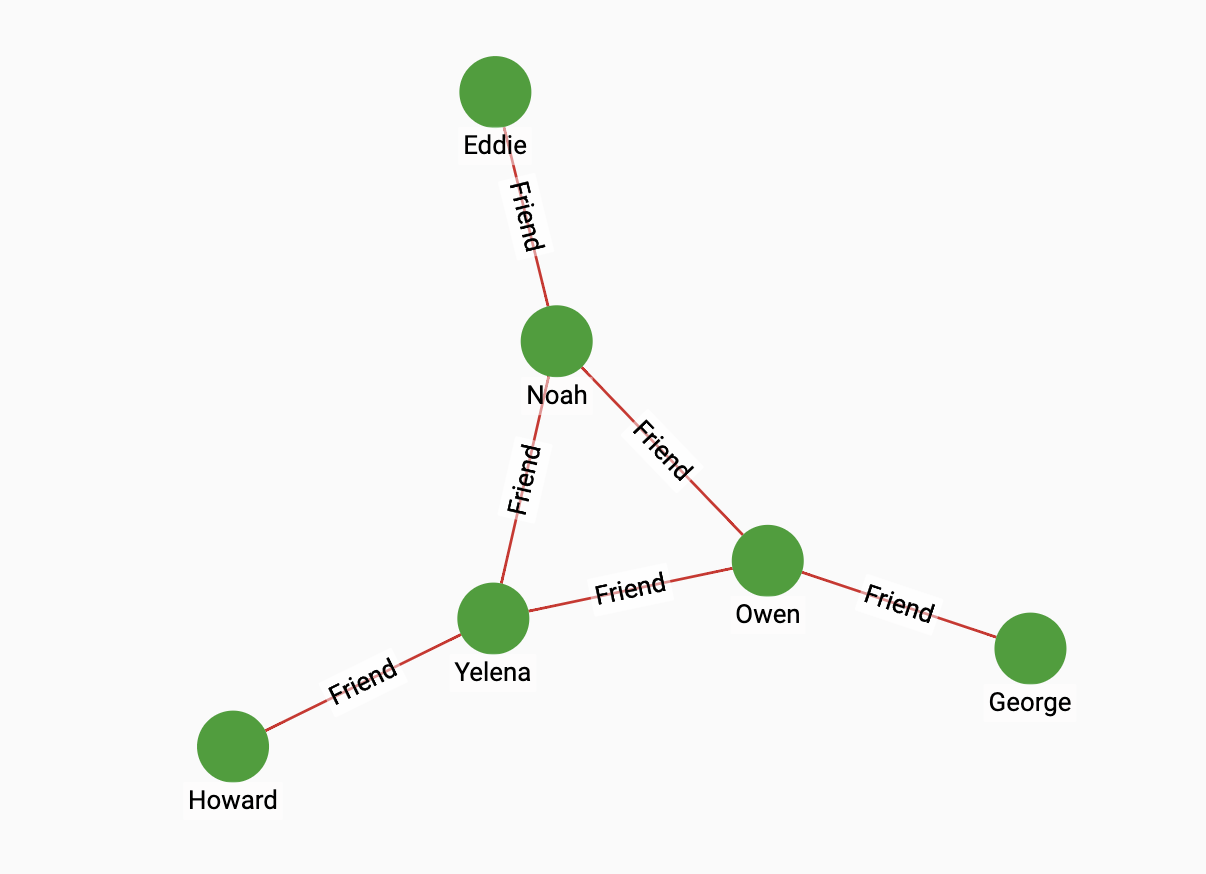# Random Walk

Random Walk is an algorithm that generate random paths in a graph. A random walk starts at every vertex that has an outgoing edge, and moves to another vertex at random. The random walk algorithm will print all possible paths of the specified size from the walks that were performed onto a file in the CSV format.

## Specifications

``````CREATE QUERY tg_random_walk(
INT step = 8, INT path_size = 4, STRING filepath = "/home/tigergraph/path.csv",
SET<STRING> edge_types, INT sample_num)``````

## Parameters

Name Description Data type

`step`

The number of hops performed in each random walk.

`INT`

`path_size`

The length of the paths to output. The length refers to the number of vertices in a path. For example, if a walk has two steps: A → B → C, then there are paths of both lengths 2 and lengths 3 that can be output from this walk. If a path size of 2 is supplied, then the algorithm outputs two paths: A → B and B → C. If the path size is 3, then there is one path: A → B → C.

`INT`

`filepath`

The filepath to output the paths to.

`STRING`

`edge_types`

The edge types that the random walk will traverse.

`SET<STRING>`

`sample_num`

At each possible step, the number of sample walks to perform.

`INT`

## Example

Suppose we have the following social graph:If we run the random walk algorithm on the graph and supply a path size of 3 and a step of 2, and a sample number of 1. Then from each vertex there is a two-step random walk, and a total of 6 three-vertex paths:

path.csv
``````Howard Yelena Noah
Noah Owen Noah
George Owen Noah
Eddie Noah Yelena
Yelena Howard Yelena
Owen George Owen``````

We can also perform a 3 step walk and still ask for three-vertex paths, in which case the number of paths would double, because in each 3 step walk, there are two possible three-vertex paths:

path.csv
``````Howard Yelena Noah
Owen Yelena Noah
Noah Owen Noah
George Owen Noah
Yelena Owen Noah
Eddie Noah Owen
Howard Yelena Noah
Noah Owen Noah
George Owen Noah
Eddie Noah Howard
Yelena Noah Howard
Owen Noah Howard``````